# Probability solutions Class 10 Maths SSC Exercise 13.1 and 13.2

SSC mathematics class 10 Probability exercise 13.1 and exercise 13.2 solutions are given.

Thsese are very easy to understand. First you study text book very well. Observe the example problems and solutions.

Observe the solutions and try them in your own method.

You can see the text book solutions for class 10

2. Sets

10. Mensuration

11. Trigonometry

13. Probability

14. Statistics

# SSC Maths solutions Probability

Chapter 13

Probability

Exercise 13.1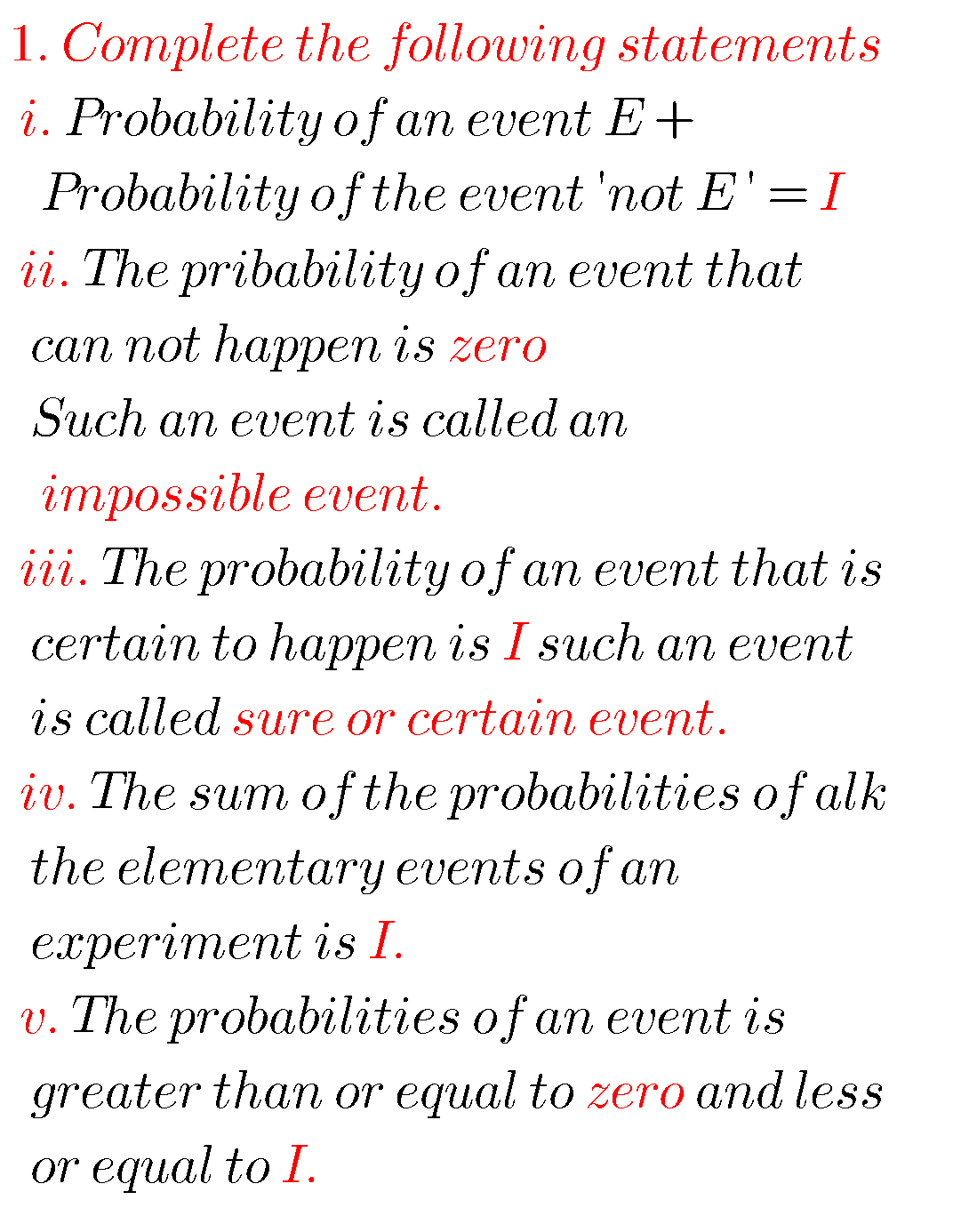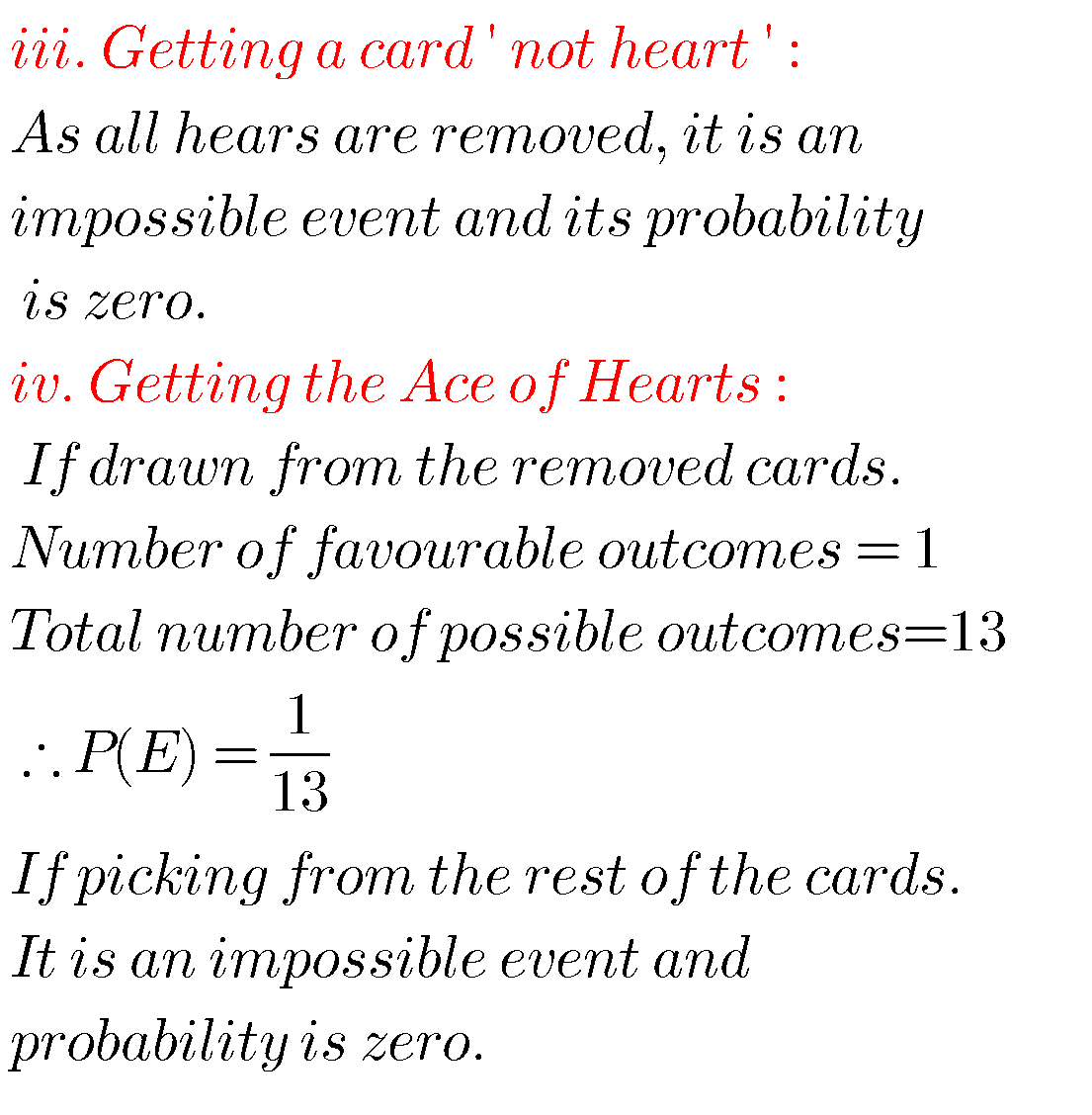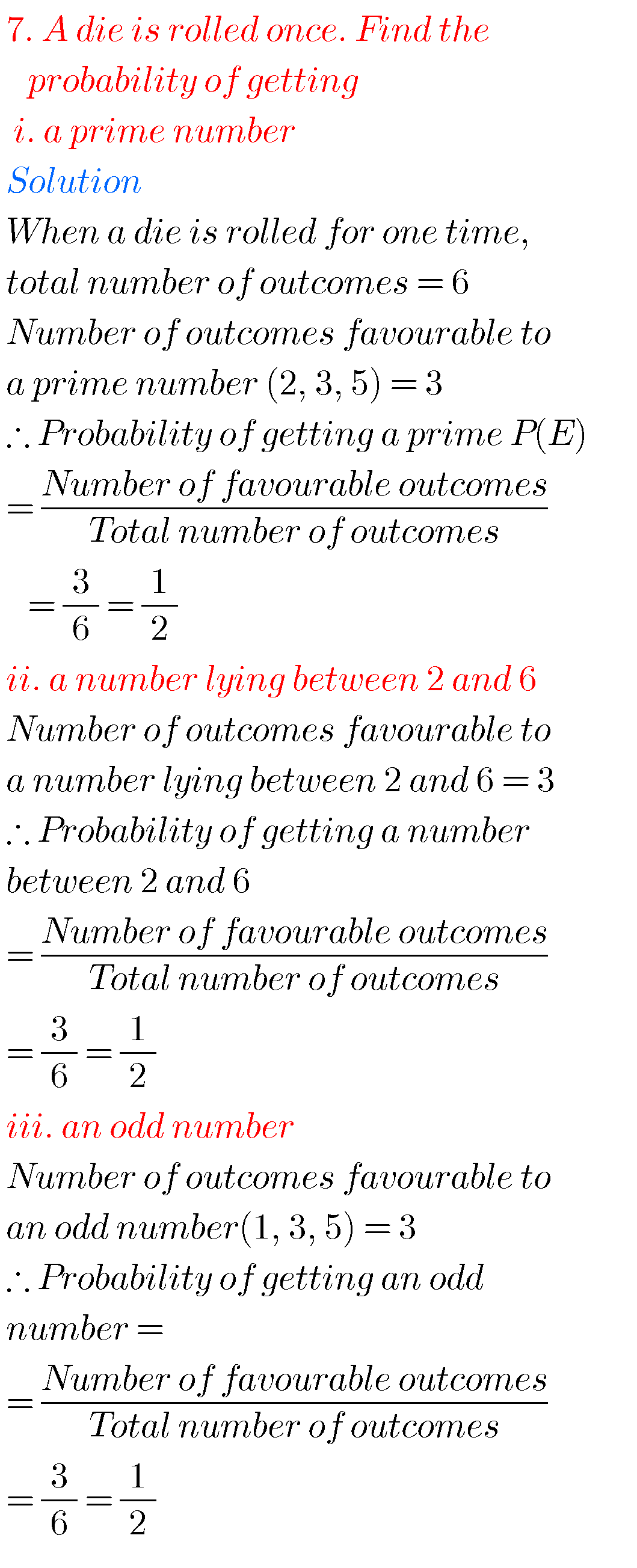## Maths solutions for Probability class 10

Exercise 13.2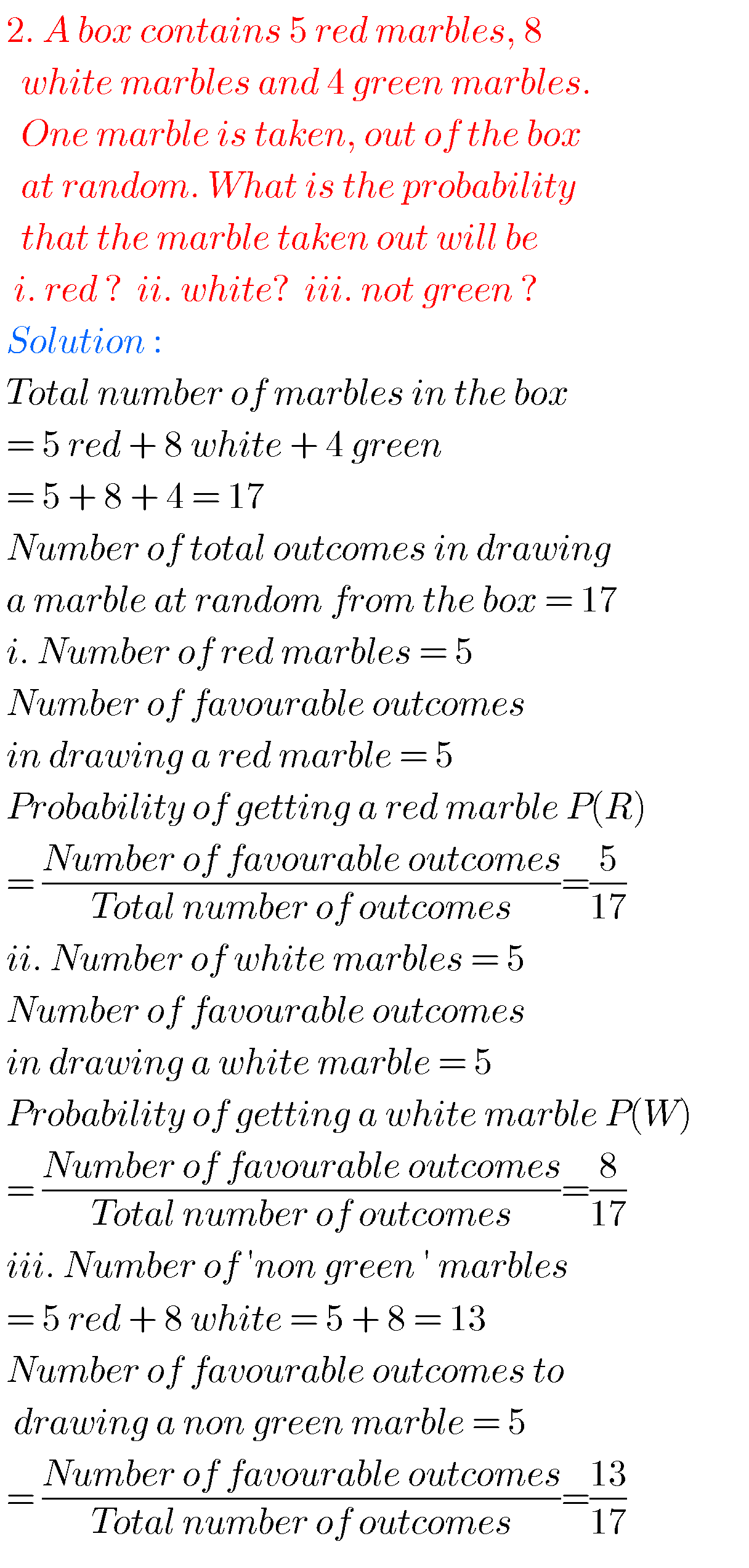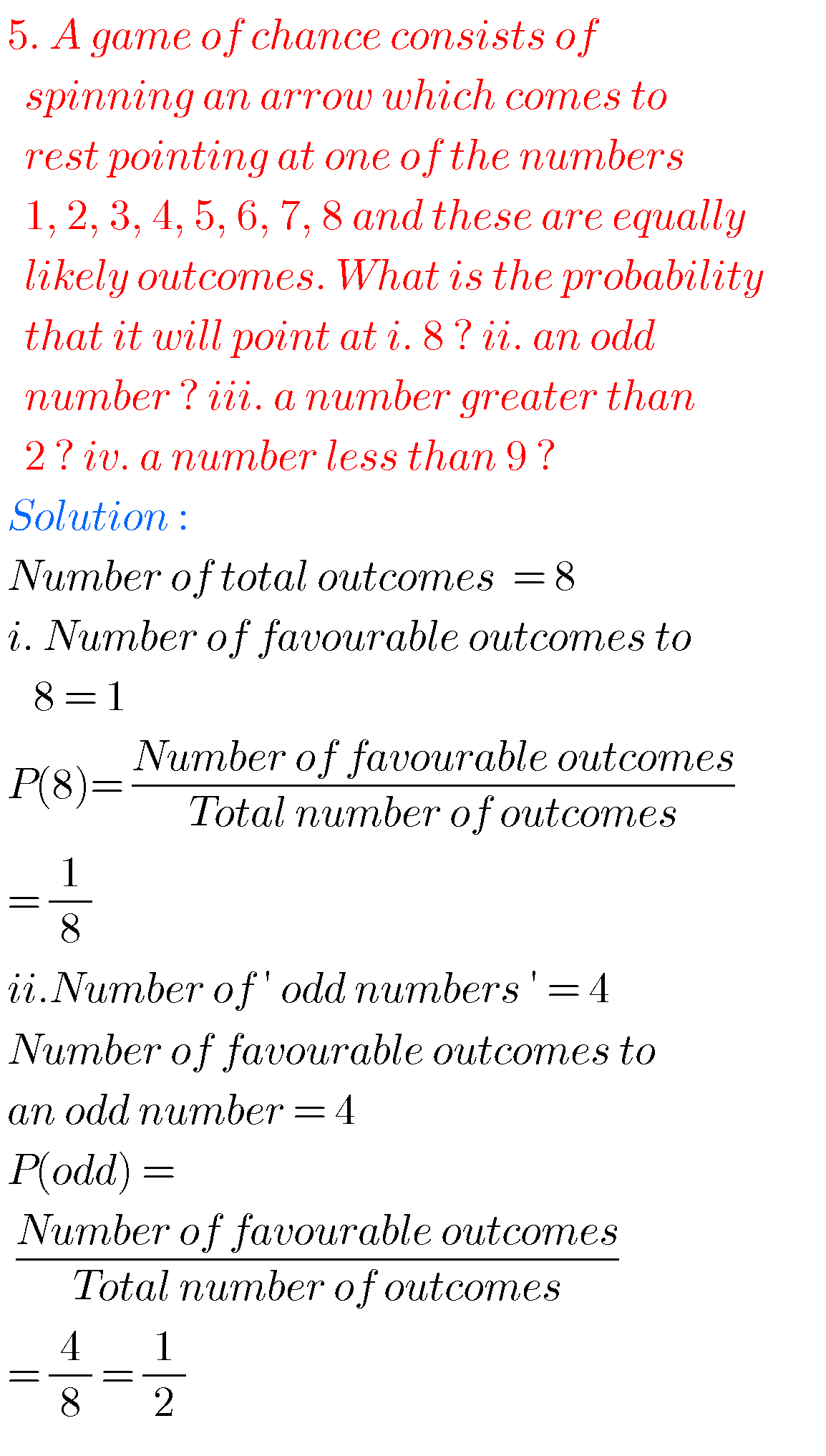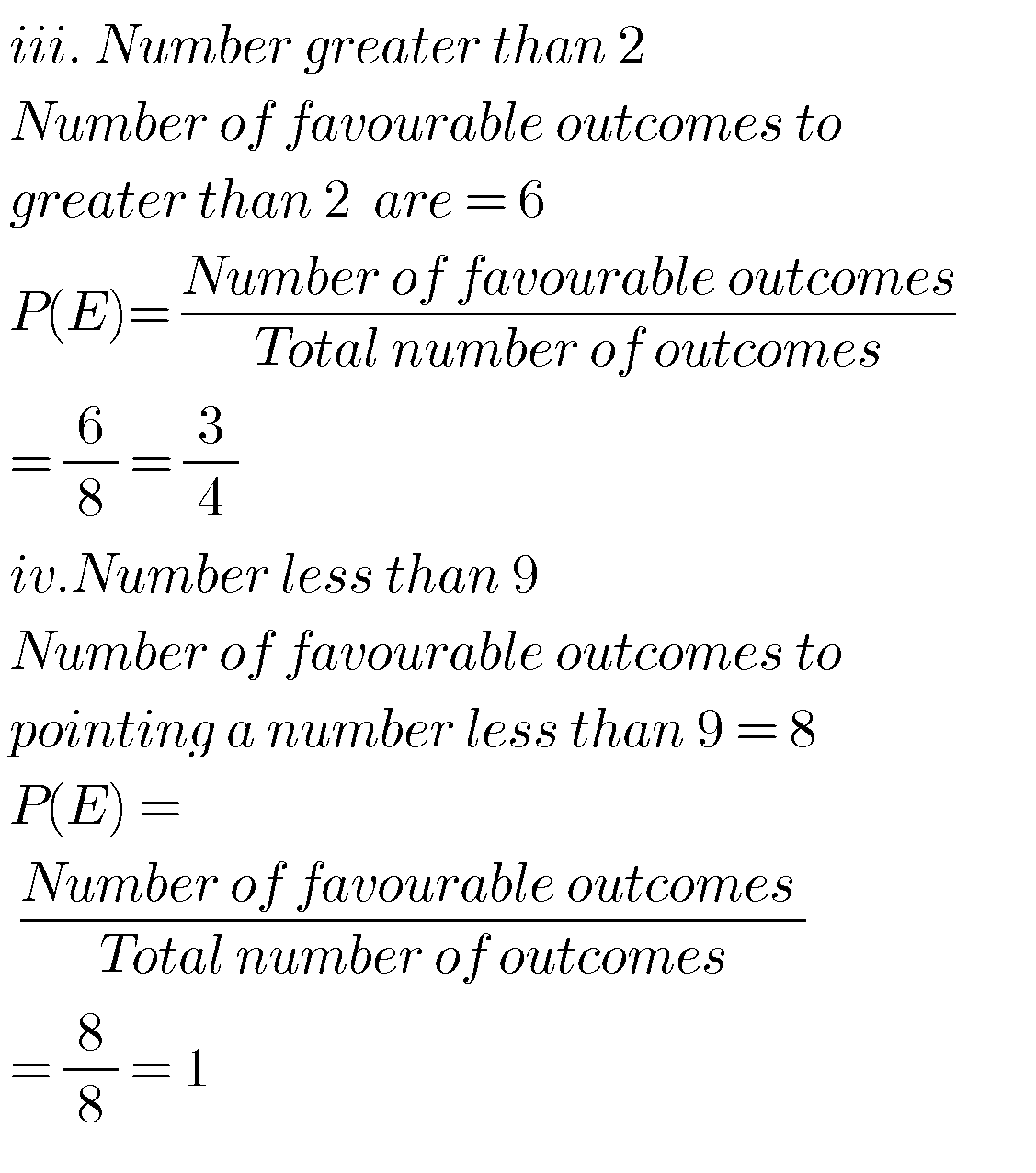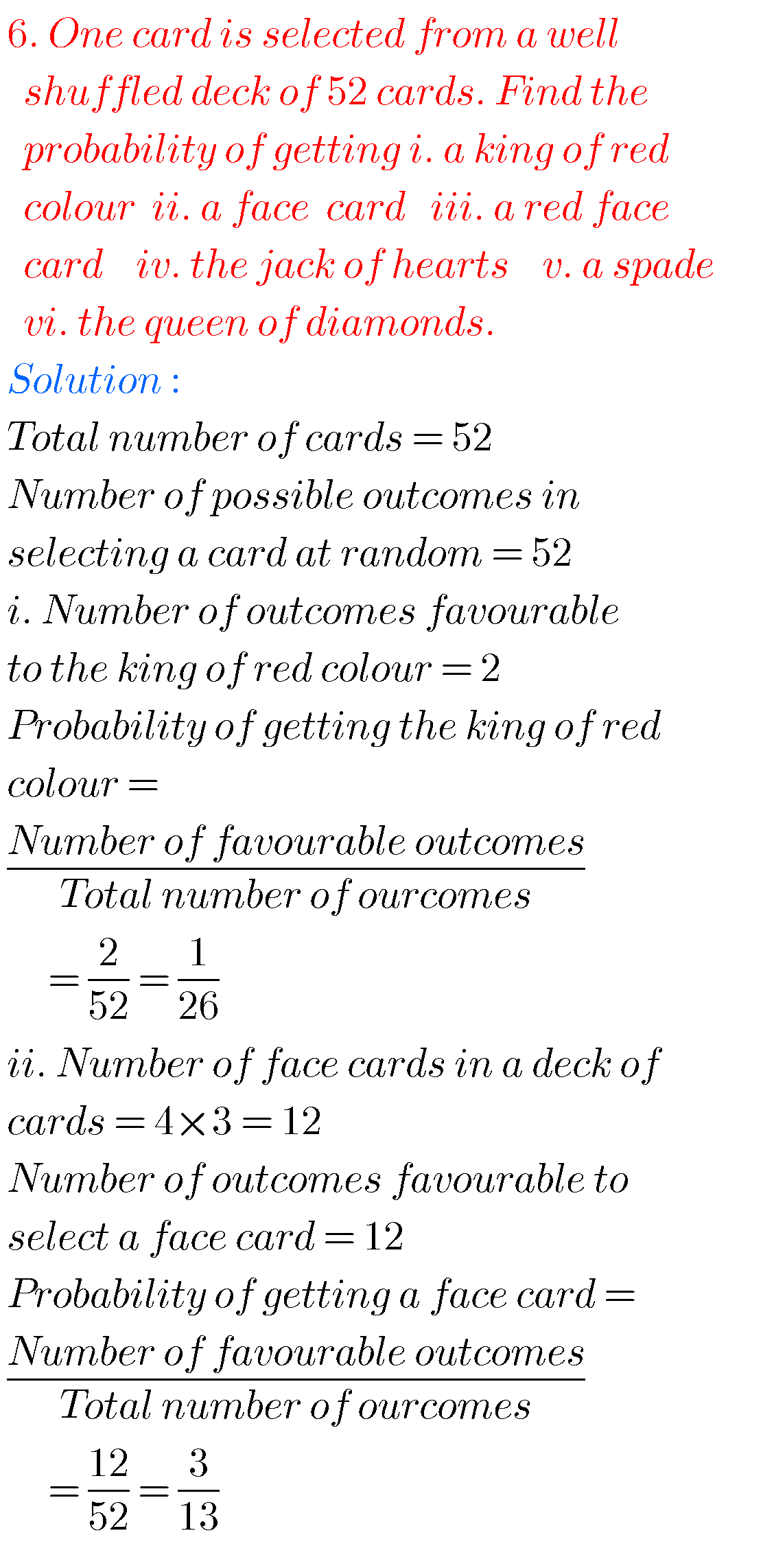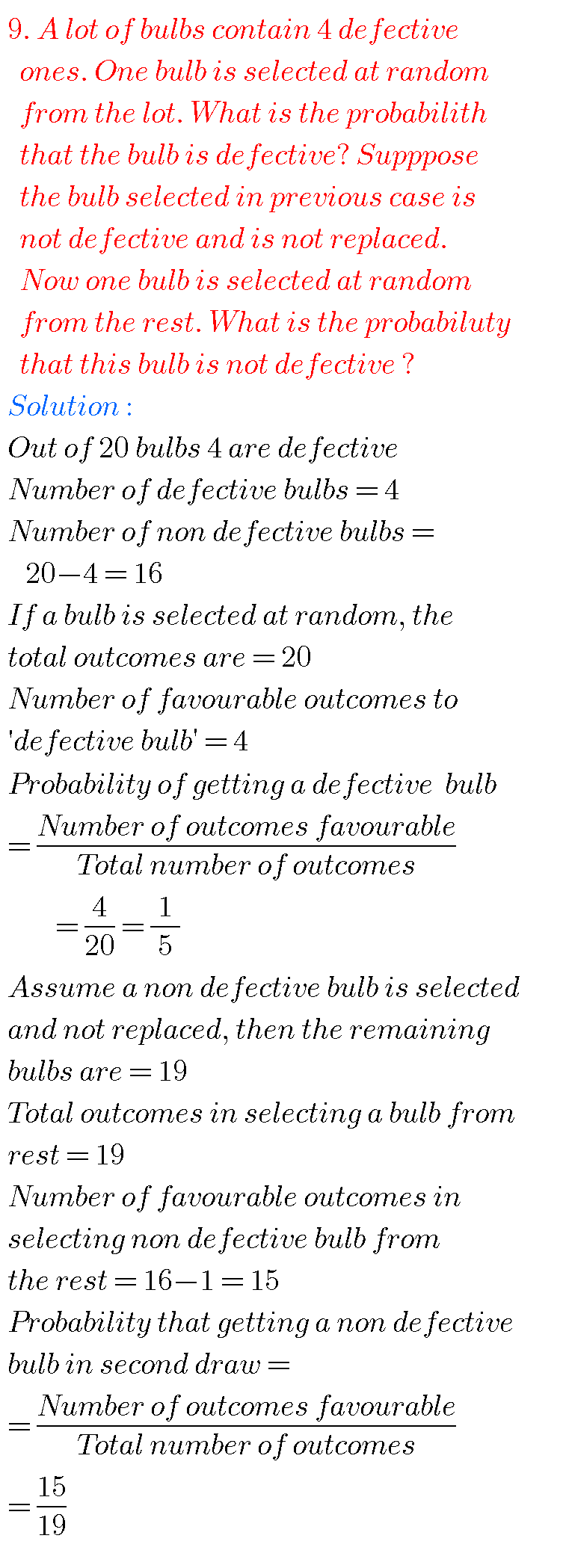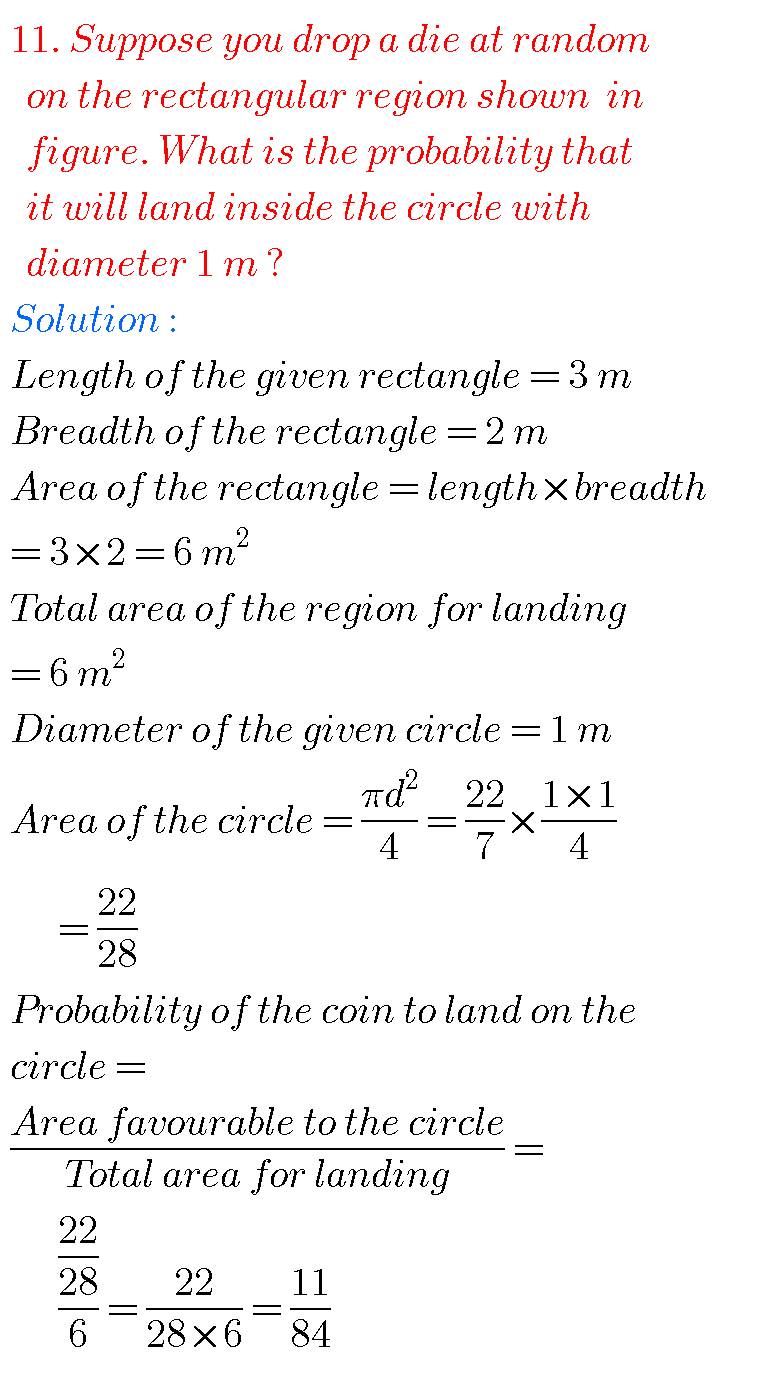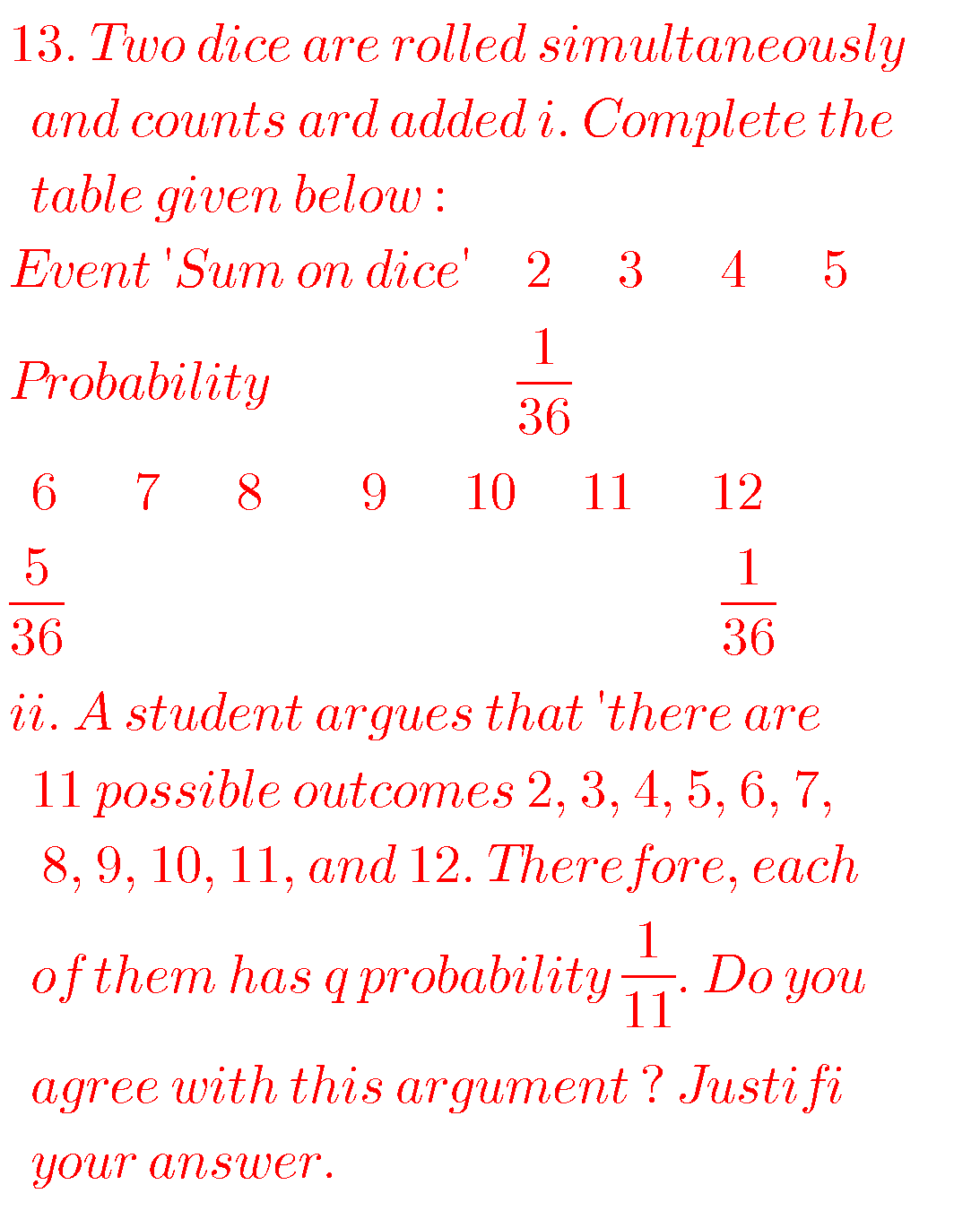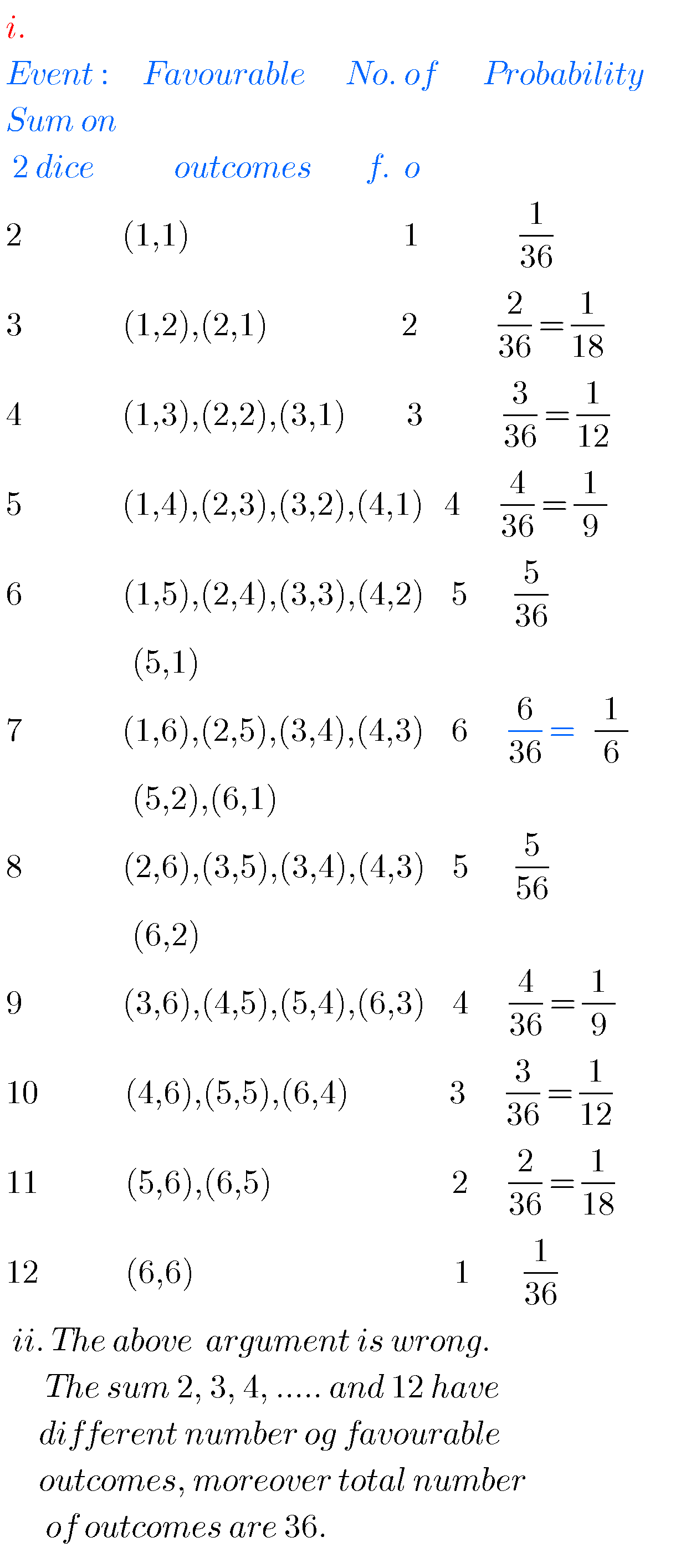Optional Exercise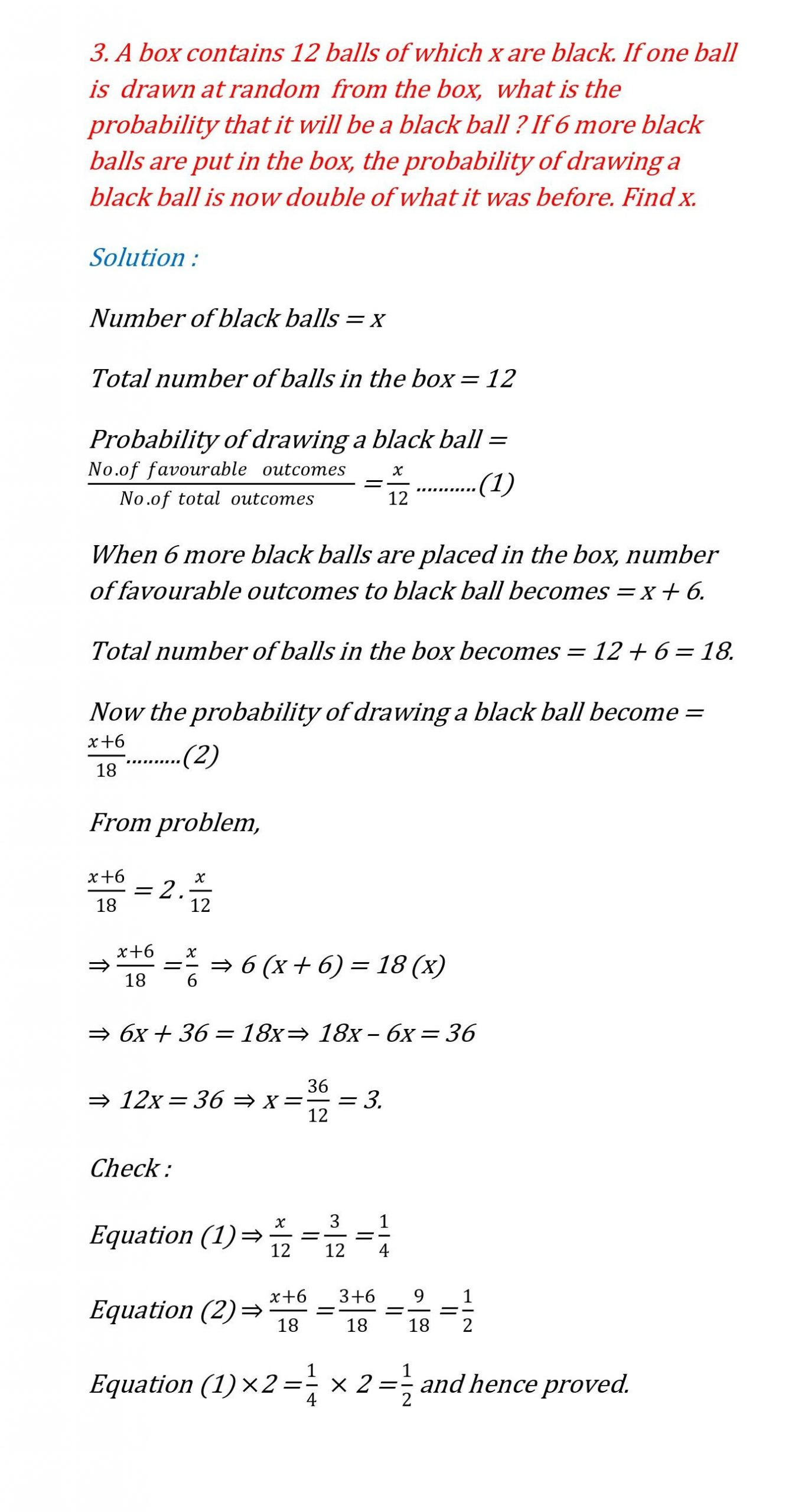Note : Observe the solutions and try them in your own method.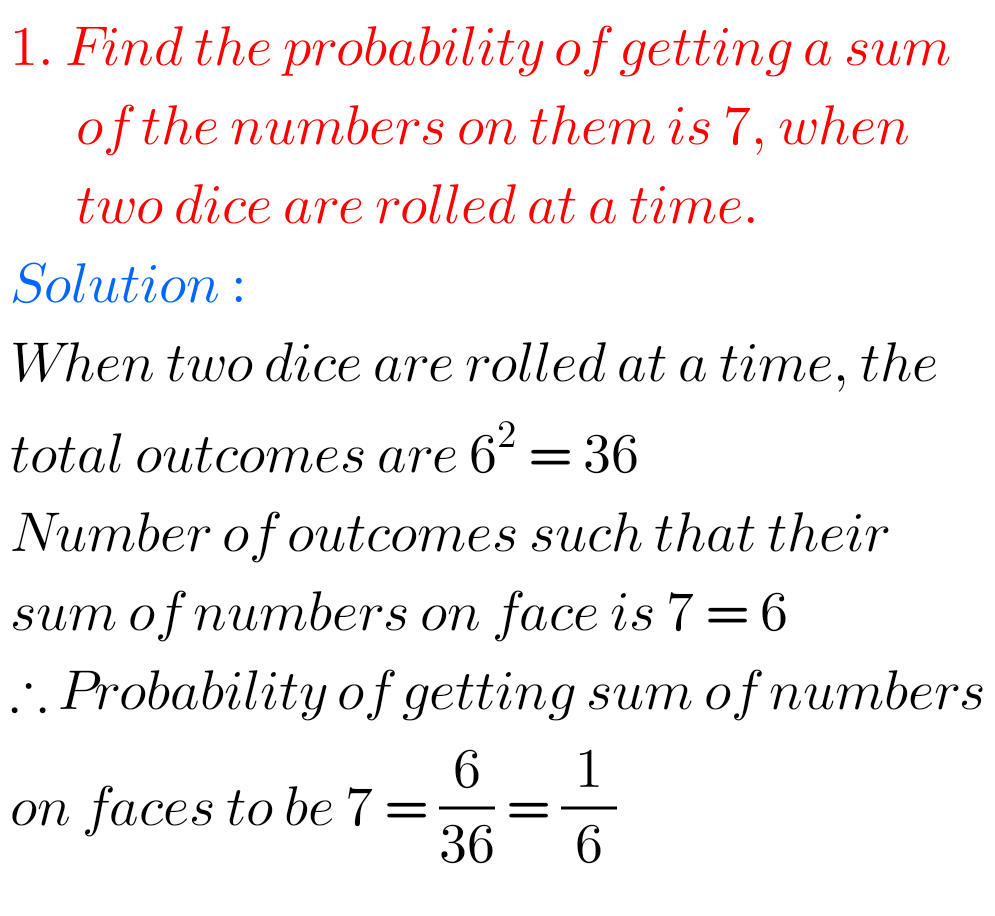You can also see the solutions

SSC Maths text book Solutions class 10

Inter maths 1A textbook solutions

Inter Maths 1A solutions

Inter Maths 1B solutions

Inter Maths 1IA text book solutions

Inter Maths IIB text book solutions

Some solutions for different classes

Ncert maths class 8 exponents and powers

Ncert maths class 7 fractions and decimals

Ncert maths class 6 playing with numbers

Ncert solutions for maths class 7 rational numbers

Ncert solutions for maths class 6 knowing our numbers

Ncert solutions for maths class 6 integers

Ncert solutions for maths class 7 integers